# H.264整数DCT公式推导及蝶形算法分析

1.为什么要进行变换

(1).可以聚集图像的能量（将能量集中到少数有意义的数值上）,如下图：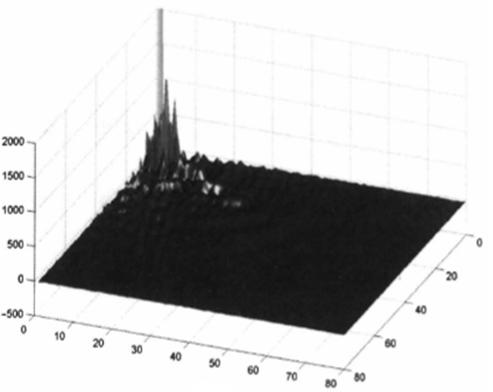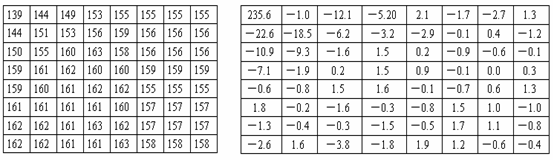1. 可以除去数据之间的相关性（以使丢掉不重要的数据对图像的质量影响很少）。
2. 变换方法应该适合用软硬件实现

2.具体变换过程

4x4的DCT变换矩阵如下: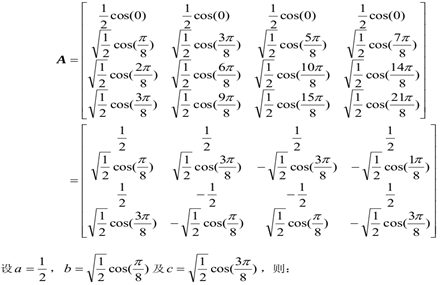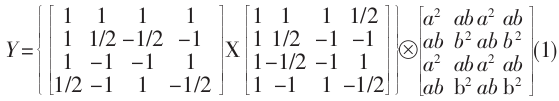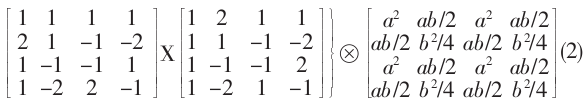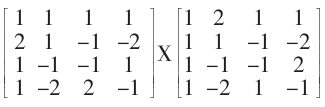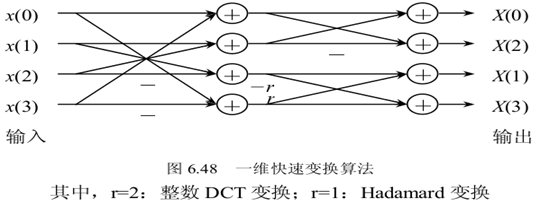X = x+x+x+x

X = 2*x+x-x-2*x

X= x-x-x+x

X = x-2x+2x-x

X = x+x+x+x

X = 2*x+x-x-2*x

X= x-x-x+x

X = x-2x+2x-x

x+x

x-x

x+x

x-x

1. 这个蝶形图和一般意义的FFT或FDCT蝶形图不同，是对H.264在整数DCT基础上的具体算法优化，只对于以上Cf矩阵。
2. 计算过程是把上面的三个4x4矩阵乘法分成两两矩阵相乘。再把残差矩阵和后来的中间结果Cf x X一行行分别输入蝶形图进行一维整数DCT计算。
3. 蝶形图优化思想就是提取矩阵的相关部分，定义中间变量，减少运算次数。

H.264中DCT变换中所使用的蝶形算法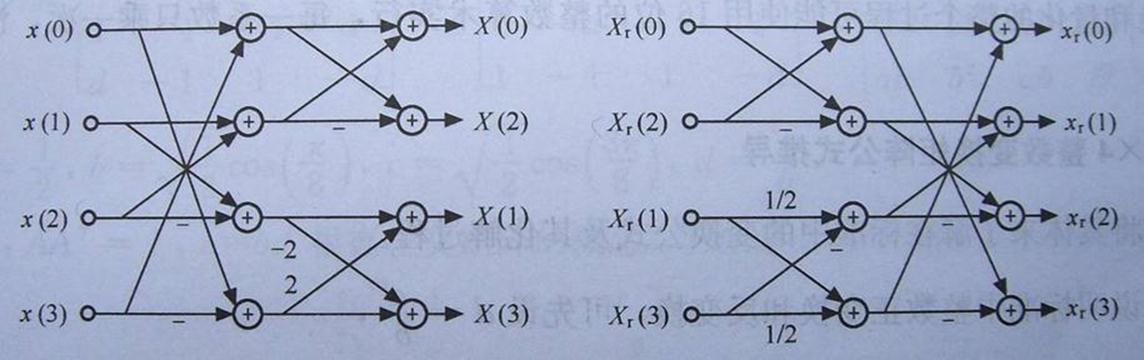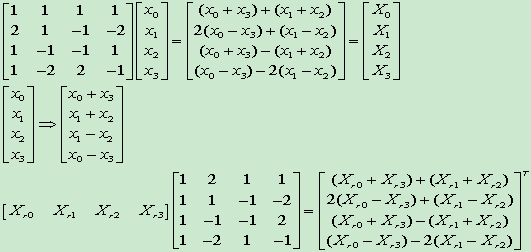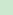// Horizontal transform水平变换，其实就是左乘Cf,
for (j=0; j < BLOCK_SIZE && !lossless_qpprime; j++)
{
for (i=0; i < 2; i++)
{
i1=3-i;
m5[i]=img->m7[i][j]+img->m7[i1][j];
m5[i1]=img->m7[i][j]-img->m7[i1][j];
}
img->m7[j]=(m5+m5);
img->m7[j]=(m5-m5);
img->m7[j]=m5*2+m5;
img->m7[j]=m5-m5*2;
}

// Vertical transform垂直变换，其实就是右乘CfT
for (i=0; i < BLOCK_SIZE && !lossless_qpprime; i++)
{
for (j=0; j < 2; j++)
{
j1=3-j;
m5[j]=img->m7[i][j]+img->m7[i][j1];
m5[j1]=img->m7[i][j]-img->m7[i][j1];
}
img->m7[i]=(m5+m5);
img->m7[i]=(m5-m5);
img->m7[i]=m5*2+m5;
img->m7[i]=m5-m5*2;

}

Xkfz007

2011年9月6日 14:09:22

posted @ 2012-07-31 14:27  Mr.Rico  阅读(9990)  评论(2编辑  收藏